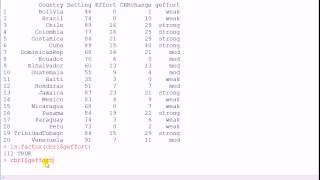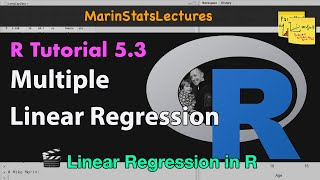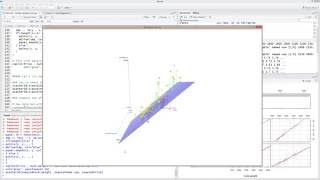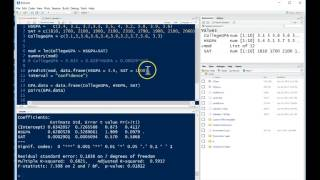R Tutorials & Resources:
Multiple Regression
 Suggest a Link Alphabetize Page Printer-Friendly List
 More R Pages: Select Page ... Getting Started with R Data Analysis with R Descriptive Statistics Creating and Transforming Variables Making Comparisons Graphing Relationships and Describing Patterns Random Assignment and Sampling Making Controlled Comparisons Foundations of Statistical Inference Hypothesis Tests with One and Two Samples Chi-Square and Analysis of Variance Correlation and Bivariate Regression Multiple Regression Analyzing Regression Residuals Logistic Regression Doing Your Own Political Analysis Advanced Political Analysis with R
Watch and Learn
Watch and Learn
Watch and Learn
Watch and Learn
 How to Change the Baseline (Reference) Category in RegressionFrom Phil Chan (5:38)Multiple Linear RegressionFrom Mike Marin (5:18)Multiple Regression with Data VisualisationFrom Jacob Cybulski (22:12)Multiple Linear Regression AnalysisFrom Katie Ann Jager (12:00)How to Change the Baseline (Reference) Category in RegressionFrom Phil Chan (5:38)Multiple Linear RegressionFrom Mike Marin (5:18)Multiple Regression with Data VisualisationFrom Jacob Cybulski (22:12)How to Change the Baseline (Reference) Category in RegressionFrom Phil Chan (5:38)Multiple Linear RegressionFrom Mike Marin (5:18)How to Change the Baseline (Reference) Category in RegressionFrom Phil Chan (5:38)Quick-R Helpful reference for using R.Tutorials & Resources
 R Short Reference Card A cheat-sheet type reference, very helpful.Tutorials & Resources
 Robust Regression with R Tutorial Alastair Sanderson shows useful methods used to handle data with outliers.Tutorials & Resources
 Swirl Stats Teaches you R programming and data science interactively, at your own pace, and right in the R consoleTutorials & Resources
 Quick-R Helpful reference for using R.Tutorials & Resources
 R Short Reference Card A cheat-sheet type reference, very helpful.Tutorials & Resources
 Robust Regression with R Tutorial Alastair Sanderson shows useful methods used to handle data with outliers.Tutorials & Resources
 Quick-R Helpful reference for using R.Tutorials & Resources
 R Short Reference Card A cheat-sheet type reference, very helpful.Tutorials & Resources
 Quick-R Helpful reference for using R.Tutorials & Resources